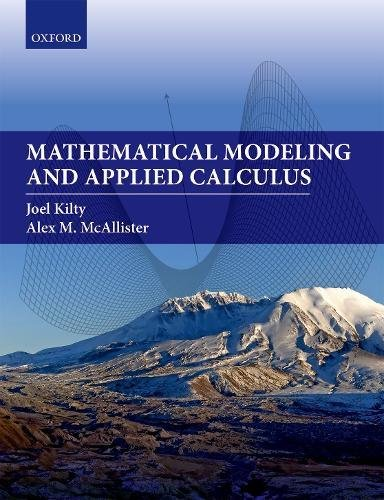# Mathematical Modeling and Applied Calculus

4.3
Description

This textbook is rich with real-life data sets, uses RStudio to streamline computations, builds “big picture” conceptual understandings, and applies them in diverse settings. Mathematical Modeling and Applied Calculus will develop the insights and skills needed to describe and model many
different aspects of our world. This textbook provides an excellent introduction to the process of mathematical modeling, the method of least squares, and both differential and integral calculus, perfectly meeting the needs of today’s students.

Mathematical Modeling and Applied Calculus provides a modern outline of the ideas of Calculus and is aimed at those who do not intend to enter the traditional calculus sequence. Topics that are not traditionally taught in a one-semester Calculus course, such as dimensional analysis and the method of
least squares, are woven together with the ideas of mathematical modeling and the ideas of calculus to provide a rich experience and a large toolbox of mathematical techniques for future studies. Additionally, multivariable functions are interspersed throughout the text, presented alongside their
single-variable counterparts. This text provides a fresh take on these ideas that is ideal for the modern student.